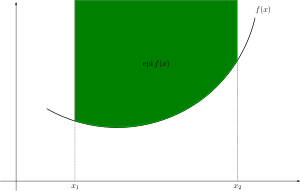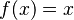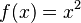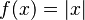# Convex function facts for kids

Kids Encyclopedia FactsA function (in black) is convex if and only if the region above its graph (in green) is a convex set.

In mathematics, a convex function is any function with value of the weighted average of 2 points is less than or equal to the weighted average of the function at those points. Also, a function is convex if and only if its epigraph is a convex set.

## Examples

Three examples are:

• A line:$f(x) = x$
• A parabola:$f(x) = x^2$
• Absolute value:$f(x) = |x|$Convex function Facts for Kids. Kiddle Encyclopedia.